# TSA Deviation and Variance VI

LabVIEW 2014 Advanced Signal Processing Toolkit Help

Edition Date: June 2014

Part Number: 372656C-01

»View Product InfoDownload Help (Windows Only)

Owning Palette: Statistical Analysis VIs

Requires: Advanced Signal Processing Toolkit

Computes the standard deviation, variance, and coefficients of variation values of a univariate or multivariate (vector) time series. Wire data to the Xt input to determine the polymorphic instance to use or manually select the instance.

Use the pull-down menu to select an instance of this VI.

 Select an instance TSA Deviation and Variance (Waveform)TSA Deviation and Variance (Array)TSA Vector Deviation and Variance (Waveform)TSA Vector Deviation and Variance (Array)

## TSA Deviation and Variance (Waveform)Xt specifies the univariate time series.weighting specifies which type of standard deviation and variance values to calculate. Options include Sample and Population. The default is Sample (N–1). Refer to the Details section for information about how this parameter affects the deviation and variance values.error in describes error conditions that occur before this node runs. This input provides standard error in functionality.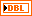standard deviation returns the standard deviation value of the input time series.variance returns the variance value of the input time series.coefficients of variation returns the coefficients of variation value of the input time series.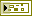error out contains error information. This output provides standard error out functionality.

## TSA Deviation and Variance (Array)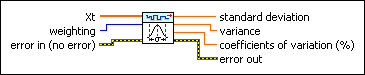Xt specifies the univariate time series.weighting specifies which type of standard deviation and variance values to calculate. Options include Sample and Population. The default is Sample (N–1). Refer to the Details section for information about how this parameter affects the deviation and variance values.error in describes error conditions that occur before this node runs. This input provides standard error in functionality.standard deviation returns the standard deviation value of the input time series.variance returns the variance value of the input time series.coefficients of variation returns the coefficients of variation value of the input time series.error out contains error information. This output provides standard error out functionality.

## TSA Vector Deviation and Variance (Waveform)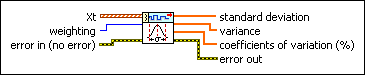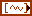Xt specifies the multivariate (vector) time series.weighting specifies which type of standard deviation and variance values to calculate. Options include Sample and Population. The default is Sample (N–1). Refer to the Details section for information about how this parameter affects the deviation and variance values.error in describes error conditions that occur before this node runs. This input provides standard error in functionality.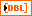standard deviation returns the vector standard deviation value of the multivariate (vector) time series.variance returns the vector variance value of the multivariate (vector) time series.coefficients of variation returns the vector coefficients of variation value of the multivariate (vector) time series.error out contains error information. This output provides standard error out functionality.

## TSA Vector Deviation and Variance (Array)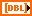Xt specifies the multivariate (vector) time series. Each column of the 2D array represents a vector at certain time.weighting specifies which type of standard deviation and variance values to calculate. Options include Sample and Population. The default is Sample (N–1). Refer to the Details section for information about how this parameter affects the deviation and variance values.error in describes error conditions that occur before this node runs. This input provides standard error in functionality.standard deviation returns the vector standard deviation value of the multivariate (vector) time series.variance returns the vector variance value of the multivariate (vector) time series.coefficients of variation returns the vector coefficients of variation value of the multivariate (vector) time series.error out contains error information. This output provides standard error out functionality.

## TSA Deviation and Variance Details

This VI calculates the standard deviation and variance values according to the following equations: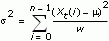where m is the arithmetic mean, s2 is variance, s is standard deviation, n is the number of time series Xt. w = n when weighting is set to Population and w = (n–1) when weighting is set to Sample.

This VI calculates the coefficients of variation according to the following equation: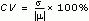## Examples

Refer to the following VIs for examples of using the TSA Deviation and Variance VI:

• Engine Knocking Monitor VI: labview\examples\Time Series Analysis\TSAApplications
• Power Line Monitor VI: labview\examples\Time Series Analysis\TSAApplications
• ARMA Prediction VI: labview\examples\Time Series Analysis\TSAGettingStarted
• Series Statistical Analysis VI: labview\examples\Time Series Analysis\TSAGettingStarted

Not Helpful